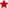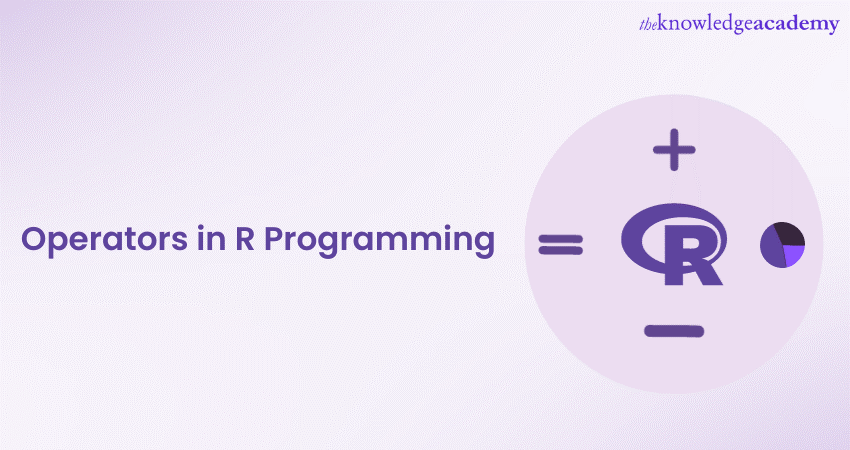## BIGGEST CYBER MONDAY SALE!

GET THE 60% EXTRA OFF!WHO WILL BE FUNDING THE COURSE?close

## Thank you for your enquiry!

One of our training experts will be in touch shortly to go over your training requirements.close

Press esc to closeBack to course information

## Thank you for your enquiry!

One of our training experts will be in touch shortly to go overy your training requirements.## Thank you for your enquiry!

One of our training experts will be in touch shortly to go over your training requirements.

### Training Outcomes Within Your Budget!

We ensure quality, budget-alignment, and timely delivery by our expert instructors.Operators in R Programming are fundamental components that allow you to perform various tasks in this powerful language known for statistical computing and data analysis. These Operators play a crucial role in executing mathematical computations. Having a good understanding of these components is necessary for tasks like value comparisons, logical evaluations, bit-level operations, and unique functionalities.

According to Statista, 4.23% developers worldwide use the R programming language. If you wish to understand the usage of Operators in this language, this blog is the right choice for you. These Operators are essential for unleashing the language's potential, making it an indispensable skill for any R programmer. Keep reading this blog to learn about Operators in R Programming, including arithmetic, logical, and many more types, each facilitating data manipulation and calculation.

1) What are Operators in R programming?

a) Arithmetic Operators

b) Assignment Operators

c) Comparison Operators

d) Logical Operators

e) Bitwise Operators

f) Special Operators

2) Conclusion

## What are Operators in R programming?

In R programming, Operators are essential elements that enable users to perform various operations on data and variables. They serve as symbols or functions that carry out specific tasks, such as mathematical computations, comparisons, logical evaluations, and bit-level operations. These Operators play a crucial role in data analysis, statistics, and other computational tasks, making them fundamental to the functionality and versatility of R programming. Some of these Operators are as follow:

Interested in becoming a programmer? Try our Programming Training today!

### Arithmetic Operators

Arithmetic Operators perform essential mathematical computations in R, enabling calculations like addition, subtraction, multiplication, division, and modulo operations. They are crucial for numeric data manipulation and form the basis of various mathematical tasks in programming.

1) Addition: This Operator (+) is used to add two or more numeric values.

2) Subtraction: This Operator (-) is used to subtract one numeric value from another.

3) Multiplication: This Operator (*) is used to multiply two or more numeric values.

4) Division: This Operator (/) is used to divide one numeric value by another.

5) Modulo: This Operator (%) is used to find the remainder after division.

 A program in R that demonstrates all arithmetic Operators # Arithmetic Operators in R Programming   # Define two numeric variables   a <- 10   b <- 3  # Addition   addition_result <- a + b   print(paste("Addition Result:", addition_result))  # Subtraction   subtraction_result <- a - b   print(paste("Subtraction Result:", subtraction_result))   # Multiplication   multiplication_result <- a * b   print(paste("Multiplication Result:", multiplication_result))   # Division   division_result <- a / b   print(paste("Division Result:", division_result))   # Modulo   modulo_result <- a %% b   print(paste("Modulo Result:", modulo_result)) Output:    "Addition Result: 13"    "Subtraction Result: 7"    "Multiplication Result: 30"    "Division Result: 3.33333333333333"    "Modulo Result: 1"

### Assignment Operators

Assignment Operators are vital for assigning values to variables in R. They simplify the process of storing and updating data, making it easier to manage and manipulate data throughout a program.

1) Assignment Operator (=): The assignment Operator, denoted with the symbol (=) is used to assign a value to a variable.

2) Shortcut assignment Operators (+=, -=, =, /=): Shortcut assignment Operators are used to perform an operation and assign the result to the variable in a single step.

 A program in R that demonstrates all assignment Operators # Assignment Operators in R Programming   # Define a variable   x <- 10   # Use the assignment Operator to update the value of x   x <- x + 5   print(paste("After using the assignment Operator, x =", x))   # Use the shortcut assignment Operators   x += 3   print(paste("After using shortcut addition, x =", x))   x -= 2   print(paste("After using shortcut subtraction, x =", x))   x *= 4   print(paste("After using shortcut multiplication, x =", x))   x /= 2   print(paste("After using shortcut division, x =", x)) Output:   "After using the assignment Operator, x = 15"    "After using shortcut addition, x = 18"    "After using shortcut subtraction, x = 16"    "After using shortcut multiplication, x = 64"    "After using shortcut division, x = 32"

### Comparison Operators

Comparison Operators are used for comparing values or expressions, generating logical outcomes (TRUE or FALSE). They play a significant role in decision-making, conditional statements, and filtering data based on specific conditions. Here’s a list of operations:

1) Equal to (==): The equal to Operator checks if two values are equal.

2) Not Equal to (!=): The not equal to Operator checks if two values are not equal.

3) Greater than (>): The greater than Operator checks if the left operand is greater than the right operand.

4) Less than (<): The less than Operator checks if the left operand is less than the right operand.

5) Greater than or Equal to (>=): The greater than or equal to Operator checks if the operand on left is greater than or equal to the operand on right.

6) Less than or Equal to (<=): The less than or equal to Operator checks if the operand on left is less than or equal to the operand on right.

 A program in R that demonstrates all comparison Operators # Comparison Operators in R Programming   # Define two variables   a <- 10   b <- 5   # Equal to (==)   result_equal <- a == b   print(paste("Equal to (a == b):", result_equal))   # Not Equal to (!=)   result_not_equal <- a != b   print(paste("Not Equal to (a != b):", result_not_equal))   # Greater than (>)   result_greater_than <- a > b   print(paste("Greater than (a > b):", result_greater_than))   # Less than (<)   result_less_than <- a < b   print(paste("Less than (a < b):", result_less_than))  # Greater than or Equal to (>=)   result_greater_equal <- a >= b   print(paste("Greater than or Equal to (a >= b):", result_greater_equal))   # Less than or Equal to (<=)   result_less_equal <- a <= b   print(paste("Less than or Equal to (a <= b):", result_less_equal)) Output:    "Equal to (a == b): FALSE"    "Not Equal to (a != b): TRUE"    "Greater than (a > b): TRUE"    "Less than (a < b): FALSE"    "Greater than or Equal to (a >= b): TRUE"    "Less than or Equal to (a <= b): FALSE"

Learn Swift and its basics with our Swift Training today!

### Logical Operators

Logical Operators are instrumental in performing logical evaluations and combining logical values to produce new results. They are fundamental for creating complex logical expressions and controlling the flow of a program. Here’s a list of operators under this section:

1) AND (&&): The AND Operator returns TRUE if both the left and right operands are TRUE.

2) OR (||): The OR Operator returns TRUE if either the left or right operand is TRUE.

3) NOT (!): The NOT Operator is used to negate the logical value of an expression.

 A program in R that demonstrates all logical Operators # Logical Operators in R Programming   # Define two logical variables   x <- TRUE   y <- FALSE   # AND (&&)   result_and <- x && y   print(paste("AND (x && y):", result_and))   # OR (||)   result_or <- x || y   print(paste("OR (x || y):", result_or))   # NOT (!)   result_not_x <- !x   result_not_y <- !y   print(paste("NOT (!x):", result_not_x))   print(paste("NOT (!y):", result_not_y)) Output:     "AND (x && y): FALSE"    "OR (x || y): TRUE"    "NOT (!x): FALSE"    "NOT (!y): TRUE"

Wish to understand R programming language in detail? Try our R Programming course!

### Bitwise Operators

Bitwise Operators are employed for low-level bit manipulation in R. They perform operations at the bit level and are typically used in specialized programming scenarios that require direct manipulation of binary data. Given below is a list of operators under this section:

1) Bitwise AND (&): The bitwise AND Operator performs a bitwise AND operation between two integers.

2) Bitwise OR (|): The bitwise OR Operator performs a bitwise OR operation between two integers.

3) Bitwise XOR (^): The bitwise XOR Operator performs a bitwise exclusive OR operation between two integers.

4) Bitwise NOT (~): The bitwise NOT Operator performs a bitwise complement operation on an integer.

5) Left Shift (<<): Using the left shift Operator lets you shifts the bits of an integer to the left.

6) Right Shift (>>): Using the right shift Operator lets you shifts the bits of an integer to the right.

 A program in R that demonstrates all bitwise Operators # Install and load the 'bitops' package   install.packages("bitops")   library(bitops)   # Define two integers   a <- 5   b <- 3   # Bitwise AND (&)   result_and <- bitAnd(a, b)   print(paste("Bitwise AND (a & b):", result_and))   # Bitwise OR (|)   result_or <- bitOr(a, b)   print(paste("Bitwise OR (a | b):", result_or))   # Bitwise XOR (^)   result_xor <- bitXor(a, b)   print(paste("Bitwise XOR (a ^ b):", result_xor))   # Bitwise NOT (~)   result_not <- bitNot(a)   print(paste("Bitwise NOT (~a):", result_not))   # Left Shift (<<)   result_left_shift <- bitShiftL(a, 2)   print(paste("Left Shift (a << 2):", result_left_shift))   # Right Shift (>>)   result_right_shift <- bitShiftR(a, 1)   print(paste("Right Shift (a >> 1):", result_right_shift)) Output:    "Bitwise AND (a & b): 1"    "Bitwise OR (a | b): 7"    "Bitwise XOR (a ^ b): 6"    "Bitwise NOT (~a): -6"    "Left Shift (a << 2): 20"    "Right Shift (a >> 1): 2"

### Special Operators

Special Operators in R, like %in% and %%, serve unique functions. %in% checks for the presence of an element in a vector, while %% is utilized for matrix multiplication. They provide specialized capabilities in data manipulation and computation tasks. Given below is a list of operators in this section:

a) %in% Operator: This Operator is used to check if an element is present in a vector.

b) %*% Operator: This Operator is used for multiplication of matrix.

 A program in R that demonstrates all special Operators # Special Operators in R Programming   # Vector membership (%in%)   vector <- c(1, 2, 3, 4, 5)   element <- 3   result_in <- element %in% vector   print(paste("Vector Membership (element %in% vector):", result_in))   # Matrix multiplication (%*%)   matrix1 <- matrix(c(1, 2, 3, 4), nrow = 2)   matrix2 <- matrix(c(5, 6, 7, 8), nrow = 2)   result_multiply <- matrix1 %*% matrix2   print("Matrix Multiplication (matrix1 %*% matrix2):")   print(result_multiply) Output:    "Vector Membership (element %in% vector): TRUE"    "Matrix Multiplication (matrix1 %*% matrix2):"   [,1] [,2]   [1,]   19   22   [2,]   43   50

## Conclusion

Operators in R Programming form the backbone of this language, empowering users to perform diverse tasks with efficiency and precision. From basic arithmetic calculations to complex data manipulations, these Operators are vital tools that elevate R's capabilities in statistical computing and data analysis, making it an invaluable language for data scientists and programmers.

Looking for a beginner friendly language? Try our Python Course today!Data Science with R Training